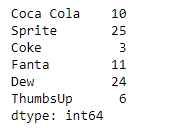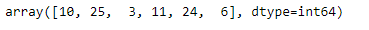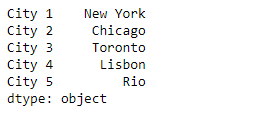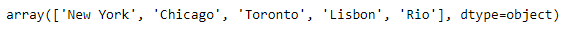# Python | Pandas Series.ravel()

• Last Updated : 11 Feb, 2019

Pandas series is a One-dimensional ndarray with axis labels. The labels need not be unique but must be a hashable type. The object supports both integer- and label-based indexing and provides a host of methods for performing operations involving the index.

Pandas` Series.ravel()` function returns the flattened underlying data as an ndarray.

Syntax: Series.ravel(order=’C’)

Parameter : order

Returns : ndarray

Example #1: Use `Series.ravel()` function to return the elements of the given Series object as an ndarray.

 `# importing pandas as pd``import` `pandas as pd`` ` `# Creating the Series``sr ``=` `pd.Series([``10``, ``25``, ``3``, ``11``, ``24``, ``6``])`` ` `# Create the Index``index_ ``=` `[``'Coca Cola'``, ``'Sprite'``, ``'Coke'``, ``'Fanta'``, ``'Dew'``, ``'ThumbsUp'``]`` ` `# set the index``sr.index ``=` `index_`` ` `# Print the series``print``(sr)`

Output :Now we will use `Series.ravel()` function to return the underlying data of the given Series object as an ndarray.

 `# return an ndarray``result ``=` `sr.ravel()`` ` `# Print the result``print``(result)`

Output :As we can see in the output, the `Series.ravel()` function has returned the an ndarray containing the data of the given series object.

Example #2: Use `Series.ravel()` function to return the elements of the given Series object as an ndarray.

 `# importing pandas as pd``import` `pandas as pd`` ` `# Creating the Series``sr ``=` `pd.Series([``'New York'``, ``'Chicago'``, ``'Toronto'``, ``'Lisbon'``, ``'Rio'``])`` ` `# Create the Index``index_ ``=` `[``'City 1'``, ``'City 2'``, ``'City 3'``, ``'City 4'``, ``'City 5'``] `` ` `# set the index``sr.index ``=` `index_`` ` `# Print the series``print``(sr)`

Output :Now we will use `Series.ravel()` function to return the underlying data of the given Series object as an ndarray.

 `# return an ndarray``result ``=` `sr.ravel()`` ` `# Print the result``print``(result)`

Output :As we can see in the output, the `Series.ravel()` function has returned the an ndarray containing the data of the given series object.

My Personal Notes arrow_drop_up